Value-Based Questions: Polynomials

# Value-Based Questions: Polynomials - Notes | Study Mathematics (Maths) Class 9 - Class 9

 1 Crore+ students have signed up on EduRev. Have you?

Q1. In a school function, students having 100% attendance are to be honoured. Class teacher of IX A gives the number of eligible students as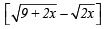whereas the class teacher of IX B gives the number of such students as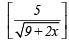If both the above numbers are equal then

(i) Find the number of prize-winners of 100% attendance in each section of class IX.
(ii) Which mathematical concept is used in the above problem?
(iii) By honouring 100% attendance holders, which value is depicted by the school administration?

(i) Number of eligible students of class IX A

=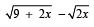Number of eligible students of class IX B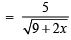Since, the number of eligible students in both sections are equal.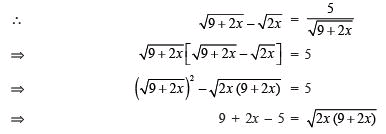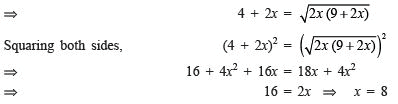Thus, in each section number of eligible students = 8
(ii) Number system.
(iii) Regularity.

Q2. Ravita donated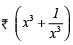to a blind school. Her friends wanted to know the amount donated by her. She did not disclose the amount but gave a hint that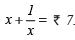(i) Find the amount donated by Ravita to the blind school.
(ii) Which mathematical concept is involved in this problem?
(iii) By donating an amount to blind school, which value is depicted by Ravita?

(i) ∵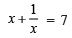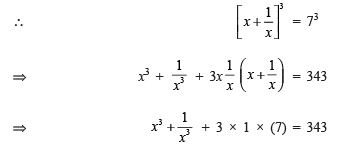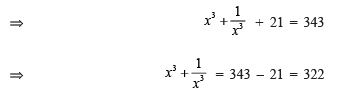Thus, the amount donated by Ravita is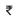322.
(ii) Polynomials.
(iii) Charity.

Q3. A group of (a + b) teachers, (a+ b2) girls and (a3 + b3) boys set out for an ‘Adult Education Mission’. If in the group, there are 10 teachers and 58 girls then:
(i) Find the number of boys.
(ii) Which mathematical concept is used in the above problem?

(iii) By working for ‘Adult Education’, which value is depicted by the teachers and students?

(i) ∵ (a + b)2 = a2 + b+ 2ab
∴ 102 = 58 + 2ab                   [∵ Number of teachers = (a + b) = 10 and Number of girls = (a2 + b2) = 58]
⇒ 100 = 58 + 2ab
⇒ 2ab = 100 – 58 = 42
⇒ ab = 42/2
= 21
Now, (a + b)3 = a3 + b3 + 3ab (a + b) ∴
(10)= a3 + b3 + 3 * 21 * 10
⇒ 1000 = a+ b+ 630 a+ b3 = 1000 – 630 = 370
∴ Number of boys = 370.
(ii) Polynomials.
(iii) Social upliftment.

The document Value-Based Questions: Polynomials - Notes | Study Mathematics (Maths) Class 9 - Class 9 is a part of the Class 9 Course Mathematics (Maths) Class 9.
All you need of Class 9 at this link: Class 9

## Mathematics (Maths) Class 9

88 videos|397 docs|109 tests
 Use Code STAYHOME200 and get INR 200 additional OFF

## Mathematics (Maths) Class 9

88 videos|397 docs|109 tests

Track your progress, build streaks, highlight & save important lessons and more!

,

,

,

,

,

,

,

,

,

,

,

,

,

,

,

,

,

,

,

,

,

;